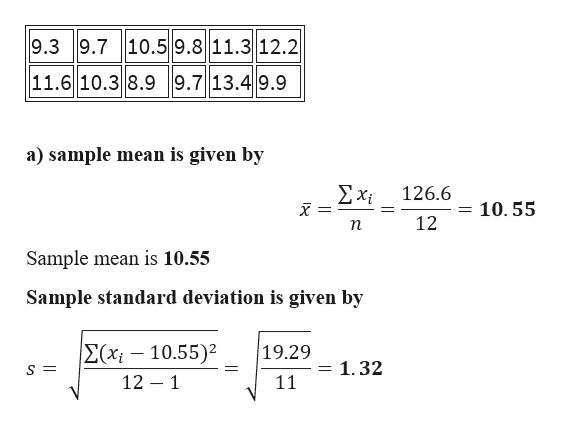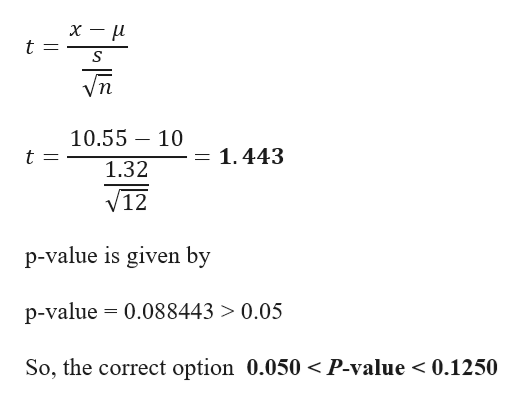Question
32 views

Carboxyhemoglobin is formed when hemoglobin is exposed to carbon monoxide. Heavy smokers tend to have a high percentage of carboxyhemoglobin in their blood.† Let x be a random variable representing percentage of carboxyhemoglobin in the blood. For a person who is a regular heavy smoker, x has a distribution that is approximately normal. A random sample of

n = 12 blood

tests given to a heavy smoker gave the following results (percent carboxyhemoglobin in the blood). Note: For degrees of freedom d.f. not in the Student's t table, use the closest d.f. that is smaller. In some situations, this choice of d.f. may increase the P-value a small amount and thereby produce a slightly more "conservative" answer.

 9.3 9.7 10.5 9.8 11.3 12.2 11.6 10.3 8.9 9.7 13.4 9.9
(a) Use your calculator to calculate
x
 x = s =

(b) A long-term population mean
μ = 10%
is considered a health risk. However, a long-term population mean above 10% is considered a clinical alert that the person may be asymptomatic. Do the data indicate that the population mean percentage is higher than 10% for this patient? Use
α = 0.05.
(i) What is the level of significance?

State the null and alternate hypotheses.
H0: μ > 10%; H1: μ = 10%H0: μ = 10%; H1: μ < 10%     H0: μ = 10%; H1: μ ≠ 10%H0: μ = 10%; H1: μ > 10%

(ii) What sampling distribution will you use? What assumptions are you making?
The Student's t, since we assume that x has a normal distribution with known σ.The standard normal, since we assume that x has a normal distribution with unknown σ.     The Student's t, since we assume that x has a normal distribution with unknown σ.The standard normal, since we assume that x has a normal distribution with known σ.

What is the value of the sample test statistic? (Round your answer to three decimal places.)

(iii) Find (or estimate) the P-value.
P-value > 0.2500.125 < P-value < 0.250     0.050 < P-value < 0.1250.025 < P-value < 0.0500.005 < P-value < 0.025P-value < 0.005

Sketch the sampling distribution and show the area corresponding to the P-value.

(iv) Based on your answers in parts (i) to (iii), will you reject or fail to reject the null hypothesis? Are the data statistically significant at level α?
At the α = 0.05 level, we reject the null hypothesis and conclude the data are statistically significant.At the α = 0.05 level, we reject the null hypothesis and conclude the data are not statistically significant.     At the α = 0.05 level, we fail to reject the null hypothesis and conclude the data are statistically significant.At the α = 0.05 level, we fail to reject the null hypothesis and conclude the data are not statistically significant.

(v) Interpret your conclusion in the context of the application.
There is sufficient evidence at the 0.05 level to conclude that the patient is asymptotic.There is insufficient evidence at the 0.05 level to conclude that the patient is asymptotic.

(c) Use the given data to find a 99% confidence interval for μ for this patient. (Round your answers to two decimal places.)
 lower limit % upper limit %
check_circle

Step 1

Given data

Sample size = 12help_outlineImage Transcriptionclose9.3 9.7 10.59.8 11.3 12.2 11.6 10.3 8.99.7 13.49.9 a) sample mean is given by Σχ 126.6 10. 55 12 п Sample mean is 10.55 Sample standard deviation is given by E(xi-10.55) 12 1 19.29 1.32 S = 1 11 fullscreen
Step 2

b)
i) Level of significance (α) = 0.05
Formulation of Hypothesis
Null Hypothesis∶H_0: μ=10%
Alternative Hypothesis:H_1:μ>10%
ii) Here the sample size is less than 30 and standard deviation is unknown, so...help_outlineImage Transcriptioncloseх — и t п 10.55 10 = 1.443 1.32 V12 p-value is given by p-value 0.088443 > 0.05 So, the correct option 0.050 < P-value < 0.1250 fullscreen

### Want to see the full answer?

See Solution

#### Want to see this answer and more?

Solutions are written by subject experts who are available 24/7. Questions are typically answered within 1 hour.*

See Solution
*Response times may vary by subject and question.
Tagged in

### Hypothesis Testing